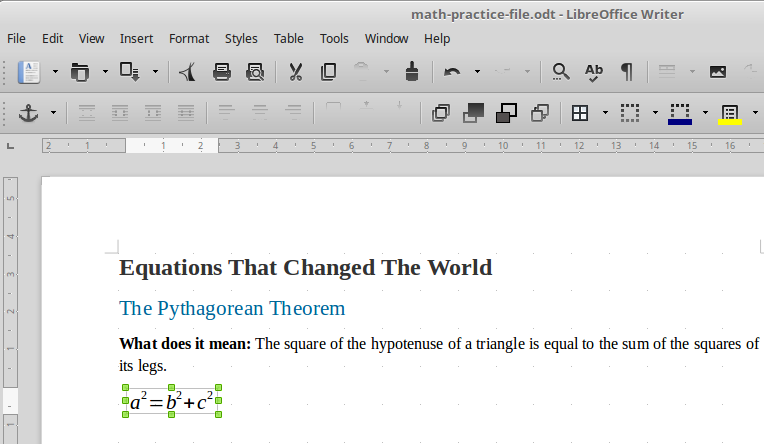## Math formula editor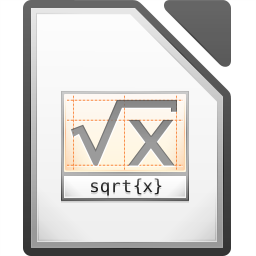LibreOffice Math is a formula editor you can use to create or edit formulas (equations) in a symbolic form. You can use Math within LibreOffice documents or as standalone application. Example formulas are shown below.Math does not carry out any actual calculation, it's just a tool to help you in typing and formating complex math formulas.

The Formula Editor in Math uses a markup language to represent formulas (like HTML). This markup language is designed to be easily read wherever possible, for example, a over b produces the fraction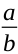when used in a formula.

You can type simple equations in Writer without using the Math component. LibreOffice Math has practical use mainly to students and professionals of Mathematics, Science and Engineering subjects. Therefore in this course will cover only the basics in Using Math.

### Using the Math formula editor

Math software works as a standalone LibreOffice application but also as a tool that integrates into other applications such as Writer.

#### As a stand alone application

You can create a formula as a separate Math document. To create a formula, start Math formula editor from LibreOffice Start Center or use the applications menu on your computer.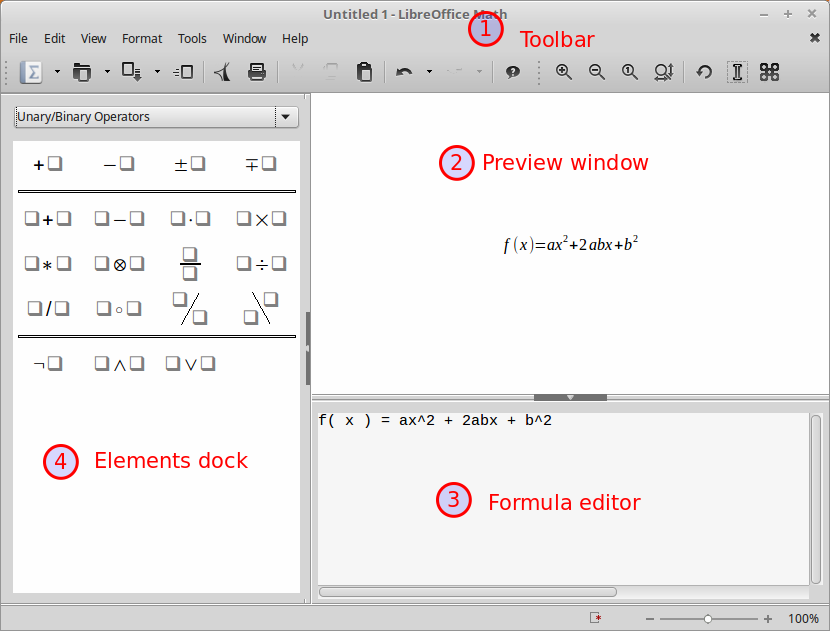As you enter the markup language in the Formula Editor, the formula will appear in the Preview. For example if you type

f(x) = ax^2 + 2abx + b^2

in the preview window the following formula will appear##### The Elements window

To make things easier for us Math provides a graphical tool to insert common math symbols to the formula editor, without having to type Math language commands. This feature is very useful especially for beginners who are not familiar with Math commands. For example to insert a fraction into a formula click on the fraction icon in the elements window, and fill the values inside the curly braces { }.The math elements are divided into categories. To find more elements change the category using the elements drop-down.

#####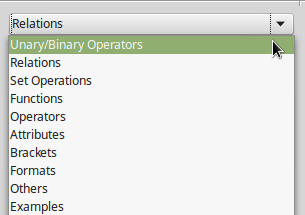Changing the view

The toolbar has buttons to control the zooming of the preview window. Click on the Optimal View button to make the formula take the optimal size.##### Saving a formula

LibreOffice’s native file format for formulas is ODF Formula, with extension *.odf. To save a formula simply click on the Save button and choose a filename (it this is the first time saving).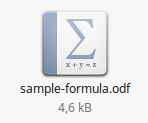### Inserting formulas into Writer

You can insert and edit a Math formulas directly into Writer. When a formula is inserted into a document, LibreOffice Writer inserts the formula into a frame and treats the formula as an OLE object.

To insert a formula, place the cursor in the position you want to insert it and use the Insert > Object > Formula menu command.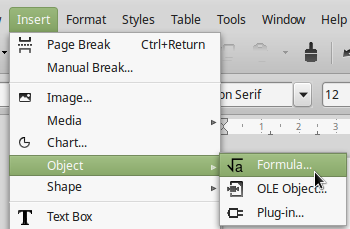Then, a window of the Math processor opens but inside the Writer environment. As you type the mathematical formula in the command window, you see a preview of the formula with the Writer document.#### Formatting the formula

While in Math editor you can format your formula using the Format submenu item. For example to change the font size choose Format > Font Size and change the Base size.